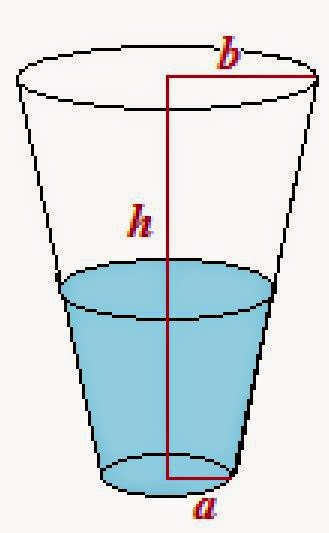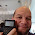## Saturday, July 12, 2014

### When is the Glass Half Full?

We have heard the famous two expressions “the glass is half-full” and the “glass is half-empty”; the former is from the view of an optimist while the latter is from the view of a pessimist.  In life, I have been both the optimist and the pessimist.

Let’s consider when a glass is actually half empty, oh sorry, half full.  This is achieved when the volume is half full when the volume of the liquid equals half of the volume of the glass.  Simple enough.

Let q be the flow rate of the liquid being poured in the glass, and assume it is a constant to keep it simple.  (Yes we can a variable flow rate q(t) – this would be appropriate when pouring viscous liquids or considering problems when we are drinking water or our favorite spirit or pop).

With V being the volume of the liquid:

dV/dt = q

dV = q dt

Taking the integral of both sides yield:

V = q*t + V0

Where V0 is the initial volume of the liquid.  Let’s assume that we start with an empty glass.  Then the liquid of the liquid inside of the glass is:

V = q*t

Let C be the volume (or the capacity) of the glass.  The glass is half full when V = C/2 or

t = C/(2 * q)

We can use this equation for various shapes of drinking glasses.  Case in point:For a cylindrical glass:  volume = π * r^2 * h

For a cylindrical glass:  C = π * r^2 * hFor a parabolic glass:  volume = π * r^2 * h/2

For a parabolic glass:  C = π * r^2 * h/2For a cup:  volume = π * h/3 * (a^2 + a*b + b^2)

For a cup:  C = π * h/3 * (a^2 + a*b + b^2)

(r, a, and b are radii – half of the distance of the diameter.)

Take the example that we have a cup with dimensions a = 1.375 in, b = 1.75 in, and h = 3.5 in.  We are going to pour iced tea in it at a flow rate of q = 8.3 in^3/sec.

C = π * 3.5/3 * (1.375^2 + 1.375 * 1.75 + 1.75^2) ≈ 26.93752 in^3

and t ≈ 26.97352/(2 * 8.3) ≈ 1.62491 sec

P.S. The flow rate I gave came from a short experiment of actually pouring Arnold Palmer Ice Tea into the glass and timing how long it takes to pour the ice tea, which it took about 3.25 seconds to fill it.  Doing the same experiment with pouring water from a bottle, which took me 7 seconds (a flow rate of approximately 3.9 in^3/sec) – but I think held the bottle closer to the cup.

Pessimists and optimists (and everyone in between) – go out and have an awesome weekend.  Cheers!

Eddie

This blog is property of Edward Shore.  2014.

#### 2 comments:

1.Great post and martini glass. The worst part about being a pessimist is when you are right :)

1.Exactly. :)

### Swiss Micros DM16L: Advanced Boolean and Factorial (up to 20)

Swiss Micros DM16L:   Advanced Boolean and Factorial (up to 20) Introduction The program listing, for the Swiss Micros DM16L and Hewlett Pac...